﻿ Graphical Interpretation of Sentences like f(x)=g(x) and f(x)>g(x)
GRAPHICAL INTERPRETATIONS OF SENTENCES LIKE $\,f(x) = g(x)\,$ and $\,f(x) \gt g(x)$
by Dr. Carol JVF Burns (website creator)
Follow along with the highlighted text while you listen!
• PRACTICE (online exercises and printable worksheets)
• Need some basic information on graphs of functions and related concepts first?
Graphs of Functions

This section should feel remarkably similar to the previous one: graphical interpretation of sentences like $\,f(x) = 0\,$ and $\,f(x) \gt 0\,$. This current section is more general—to return to the previous ideas, just let $\,g(x)\,$ be the zero function.

If you know the graphs of two functions $\,f\,$ and $\,g\,$,
then it is very easy to visualize the solution sets of sentences like $\,f(x) = g(x)\,$ and $\,f(x)\gt g(x)\,$.
This section shows you how!

A key observation is that a sentence like $\,f(x) = g(x)\,$ or $\,f(x) \gt g(x)\,$ is a sentence in one variable, $\,x\,$.
To solve such a sentence, you are looking for value(s) of $\,x\,$ that make the sentence true.
The functions $\,f\,$ and $\,g\,$ are known, and determine the graphs that you'll be investigating.

Recall that the graph of a function $\,f\,$ is a picture of all points of the form $\,(x,f(x))\,$,
and the graph of a function $\,g\,$ is a picture of all points of the form $\,(x,g(x))\,$.

In particular, the $\,y$-value of the point $\,(x,f(x))\,$ is the number $\,f(x)\,$
and the $\,y$-value of the point $\,(x,g(x))\,$ is the number $\,g(x)\,$.

If $\,f(x)\gt g(x)\,$, then the point $\,(x,f(x))\,$ lies above the point $\,(x,g(x))\,$.
If $\,f(x)=g(x)\,$, then the graphs of $\,f\,$ and $\,g\,$ intersect at this point.
If $\,f(x)\lt g(x)\,$, then the point $\,(x,f(x))\,$ lies below the point $\,(x,g(x))\,$.

These concepts are illustrated below.

The notation $\,P(x,f(x))\,$ is a convenient shorthand for:   the point $\,P\,$ with coordinates $\,(x,f(x))$

 $P_1(x,f(x))\,$ and $\,P_2(x,g(x))\,$ with $\,f(x)\gt g(x)\,$ $P_1(x,f(x))\,$ and $\,P_2(x,g(x))\,$ with $\,f(x)=g(x)$ $P_1(x,f(x))\,$ and $P_2(x,g(x))\,$ with $\,f(x)\lt g(x)$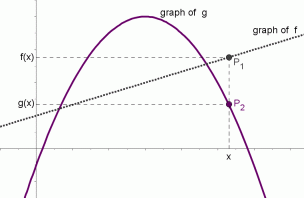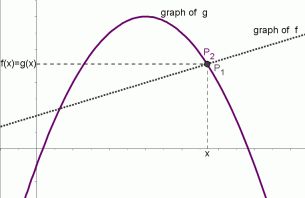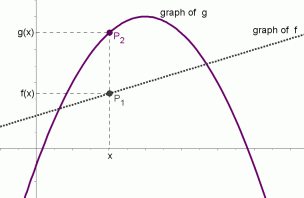for this value of $\,x\,$, the graph of $\,f\,$ lies above the graph of $\,g$ for this value of $\,x\,$, the graphs of $\,f\,$ and $\,g\,$ intersect for this value of $\,x\,$, the graph of $\,f\,$ lies below the graph of $\,g$
 The graphs of functions $\,f\,$ and $\,g\,$ are shown at right. The solution set of the inequality ‘$\,f(x)\gt g(x)\,$’ is shown in green. It is the set of all values of $\,x\,$ for which the graph of $\,f\,$ lies above the graph of $\,g\,$.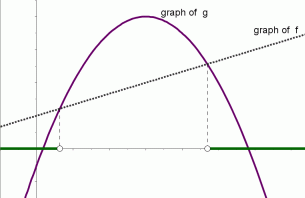The graphs of functions $\,f\,$and $\,g\,$ are shown at right. The solution set of the equation ‘$\,f(x)=g(x)\,$’ is shown in green. It is the set of all values of $\,x\,$ for which the graphs of $\,f\,$ and $\,g\,$ intersect.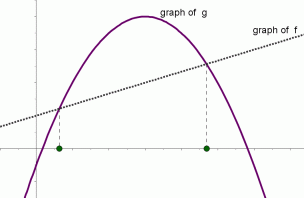The graphs of functions $\,f\,$ and $\,g\,$ are shown at right. The solution set of the inequality ‘$\,f(x)\lt g(x)\,$’ is shown in green. It is the set of all values of $\,x\,$ for which the graph of $\,f\,$ lies below the graph of $\,g\,$.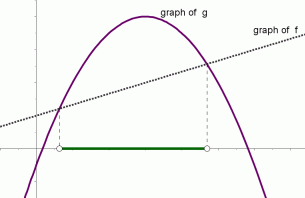The graphs of functions $\,f\,$ and $\,g\,$ are shown at right. The solution set of the inequality ‘$\,f(x)\ge g(x)\,$’ is shown in green. It is the set of all values of $\,x\,$ for which the graph of $\,f\,$ lies on or above the graph of $\,g\,$.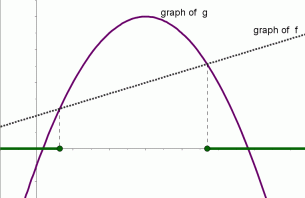The graphs of functions $\,f\,$and $\,g\,$ are shown at right. The solution set of the inequality ‘$\,f(x)\le g(x)\,$’ is shown in green. It is the set of all values of $\,x\,$ for which the graph of $\,f\,$ lies on or below the graph of $\,g\,$.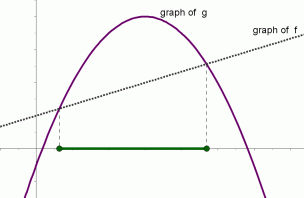EXAMPLE:

The graphs of two functions, each with domain $\,\mathbb{R}\,$, are shown below:
$\,f\,$ is a parabola, shown in the black dotted pattern;
$\,g\,$ is a cubic polynomial, shown in purple.
These two curves intersect at the points $\,(-1,2)\,$, $\,(0,0.5)\,$ and $\,(1,1)\,$.

Pay attention to the difference between the brackets   ‘$\,[\ ]\,$’   and parentheses   ‘$\,(\ )\,$’   and braces   ‘$\,\{\ \}\,$’   in the solutions sets!

Remember that the symbol ‘$\,\cup\,$’, the union symbol, is used to put sets together.The solution set of the inequality ‘$\,f(x)\gt g(x)\,$’ is:   $\,(-\infty ,-1) \cup (0,1)$ The solution set of the equation ‘$\,f(x)=g(x)\,$’ is:   $\,\{-1,0,1\}\,$ The solution set of the inequality ‘$\,f(x)\lt g(x)\,$’ is:   $\,(-1,0)\cup (1,\infty)\,$ The solution set of the inequality ‘$\,f(x)\ge g(x)\,$’ is:   $\,(-\infty ,-1] \cup [0,1]$ The solution set of the inequality ‘$\,f(x)\le g(x)\,$’ is:   $\,[-1,0] \cup [1,\infty )$
Let's discuss the solution set of the inequality ‘$\,f(x)\gt g(x)\,$’.

Imagine a vertical line passing through the graph, moving from left to right.
Every time the vertical line is at a place where the graph of $\,f\,$ lies above the graph of $\,g\,$,
then you must include that $\,x$-value in the solution set.
Be extra careful of places where something interesting is happening
(like where the graphs intersect).
Master the ideas from this section